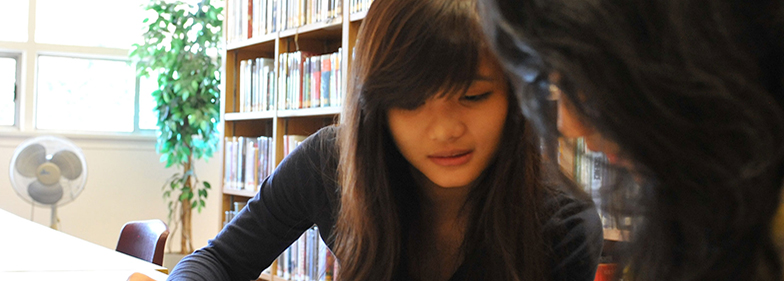## FIRST-YEAR CALCULUS TUTORING### Overview

First-year calculus in most post-secondary institutions are divided into 2 courses or semesters: differentiation (Calculus I) and integration (Calculus II).

### Post-Secondary Institutions We Have Served

We provide tutoring services for all post-secondary institutions across the Lower Mainland. Our tutors have assisted students from the following institutions:

• UBC
• SFU
• BCIT
• Capilano University
• Columbia College
• Corpus Christi College
• Douglas College
• Kwantlen Polytechnic University
• Langara College
• St. Mark's College

### Courses We Have Tutored

UBC SFU Langara KPU
MATH 100 MATH 151 MATH 1152 MATH 1120
MATH 101 MATH 152 MATH 1153 MATH 1130
MATH 102 MATH 154 MATH 1171 MATH 1140
MATH 103 MATH 155 MATH 1173 MATH 1220
MATH 104 MATH 157 MATH 1174 MATH 1230
MATH 105 MATH 158 MATH 1183 MATH 1240
MATH 110 MATH 1271
MATH 180 MATH 1273
MATH 184 MATH 1274
MATH 1283

### Differentiation (Calculus I) Topics

• Limits and Continuity
• Derivative of a Function
• Product and Quotient Rule
• Chain Rule
• Implicit Differentiation
• Related Rates
• Linear Approximation and Differentials
• Optimization Problems
• Exponential Growth, Decay, and Compound Interest

### Integration (Calculus II) Topics

• Limits and Continuity
• Derivative of a Function
• Product and Quotient Rule
• Chain Rule
• Implicit Differentiation
• Related Rates
• Linear Approximation and Differentials
• Optimization Problems
• Exponential Growth, Decay, and Compound Interest

### How Math Tutor Network can help?

Math Tutor Network has specialized calculus tutors. Tutors may be TA's from university who have previously teaching experience in first-year calculus, experts in the field, or teachers with a solid calculus background.

### Tutoring Lessons

For students who only require help on homework problems and for test preparation, Math Tutor Network recommends one 2-hour lesson per week.

For students having difficulties understanding the concepts the teacher go over in class, Math Tutor Network recommends two or more 2-hour lessons per week.